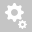##Recently viewed tickets# Question about Non Linear Analysis with FEA

Question:

Hi all,

For the model A, Specified Displacement is assigned with the 'Initial Stiffness' method as an iteration method.I got the load-displacement curve as shown below, which looks reasonable.For the model B, horizontal loads are applied with the 'Arc-Length' method.I got the load-displacement curve as shown below.I don't understand why the softening behavior after peak is not obtained because I used the 'Arc-Length' method.

Thanks

Hi,

Here is the explanation.
This model is very sensitive so that a small increment of load can lead to the sudden change of response. Thus, the increment of load should be small enough in order to get true behavior of the structure.
For the 'Arc Length' method, we can reduce the increment of load by reducing 'Initial Load Factor'. We have reset the analysis control as shown below.We were able to get the response even after peak as shown below although the analysis could not be converged after peak because the structure has lost the resistance.Redraw up to 16 mm.Zoom in the area circled in red in the above graph.As you see in the above graph, the load is decreased at 1.3 mm of displacement and increased back, which can be simulated because we used the 'Arc Length' method.

You have obtained the following response from the specified displacement with the 'Initial Stiffness' iteration method.
- Number of load step: 1000, Displacement norm = 0.01, In this setting, the load increment per step at the beginning is about 68000 N.Even with the displacement control model with 'Specified Displacement' load, the analysis is not converged after peak if we reduce the load increment and the displacement norm to 3400 N and 0.005, respectively.In summary, it was difficult to obtain the response after peak because of the two reasons:
1) The structure becomes unstable suddenly after peak, which leads to the divergence of analysis.
2) The load increment is not small enough.

Regards,
DK

Creation date: 12/21/2018 2:19 AM (dklee@midasit.com)      Updated: 12/21/2018 2:13 PM (dklee@midasit.com)